The analysis of optimal punishment in this chapter can be put in more precise mathematical form as follows. We define: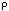(b): the density of offenses per year as a function of the gain b to the offender of committing the offense.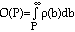: the number of offenses per year whose perpetrators gain more than P by committing them. Since an offense will be committed only if the gain is at least as great as the expected punishment, O(P) is the number of offenses that occur annually if the expected punishment is P.

C(P): the cost per offense of imposing an expected punishment P, using the least costly combination of actual punishment and probability. I assume that this does not depend on the number of offenses.

D: the damage done per offense. For simplicity this too is assumed independent of the number of offenses.

We wish to find, the expected punishment which minimize a social cost function:

SC(P) = O(P) [D+C(P)] &endash;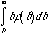(Equation 1)

=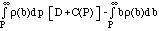The first term on the right hand side is the cost of crime, the number of offenses multiplied by damage per offense plus enforcement cost (the cost of catching, convicting, and punishing offenders) per offense. The second term is the benefit of offenses to the offenders. The integral starts at b=P because only crimes for which benefit is at least equal to expected punishment will be committed.

Setting the derivative of SC(P) with regard to P equal to 0, we have, for P equal to its optimum value :

0 = - D()+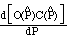+() =()[-D] +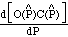Solving for the optimal punishment we have: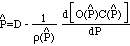(Equation 2)

Equation 2 is the mathematical equivalent of the result derived in the earlier verbal argument. O(P)C(P) is the total cost of imposing an expected punishment of P on Q(P) offenses. Deterring one more offense requires an increase in P of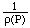, so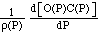is the cost of deterring one more offense. If>0 at P=, then total enforcement cost is increasing with increasing punishment and, as can be seen from Equation 2, the optimal punishment is less than the damage done. If <0 at P= , then total enforcement cost is decreasing with increasing punishment (due to the decrease in the number of offenses) and the optimal punishment is more than the damage done. If<0 at P=, then total enforcement cost is decreasing with increasing punishment (due to the decrease in the number of offenses) and the optimal punishment is more than the damage done.